Home > CCA2 > Chapter 10 > Lesson 10.3.1 > Problem10-161

10-161.
1. For the function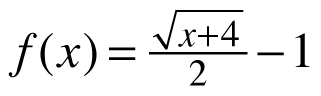, complete parts (a) through (d) below. Homework Help ✎

1. Sketch the graph and the inverse.

2. Find the equation of the inverse function.

3. Determine the domain and range of the inverse.

4. Compute f −1(f(5)).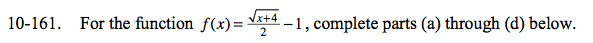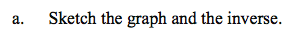If you are stuck trying to sketch the graph, make a table.
Remember that to get an inverse, interchange the x- and y-values.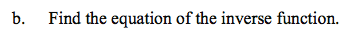$\text{Solve for } y\text{: } x=\frac{\sqrt{y+4}}{2}-1$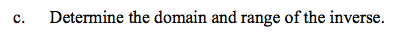Look at your graphs in part (a).
What are the smallest and largest possible values of x?
What are the samllest and largest possible values of y?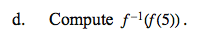5